# Catch up on the latest AI articles# Can Robots Trained By Deep Reinforcement Learning Be Used In Factories For Assembly Tasks?

3 main points
✔️ Evaluated the RL algorithm on a large scale on the NIST assembly benchmark and showed a success rate of 99.8% out of 13K trials
✔️ We proposed a framework called SHIELD with modifications to DDPGfD.
✔️ We compared the performance with humans on two more difficult tasks and showed the usefulness of RL.

written by Jianlan LuoOleg SushkovRugile PevceviciuteWenzhao LianChang SuMel VecerikNing YeStefan SchaalJon Scholz
(Submitted on 21 Mar 2021 (v1), last revised 31 Jul 2021 (this version, v4))

Subjects: Artificial Intelligence (cs.AI); Robotics (cs.RO)code：The images used in this article are from the paper, the introductory slides, or were created based on them.

## first of all

Currently, robots are used in factories for the task of inserting parts into products, which can be solved by programming the robot's skills in advance in a constrained environment. However, the time and cost required for this programming are very high, which makes it difficult to apply robots to products that are produced in small quantities, even if they are used in large-scale production.

Deep reinforcement learning, on the other hand, has been attracting attention in recent years because it is becoming possible to learn the skills needed to solve a task with less engineering. However, despite various researches, it is considered that it is not at a level where it can be used in industry, and there is no benchmark to measure whether it can be used in industry or not. The NIST assembly benchmark, which is shown in the figure below, has been attracting attention recently because it provides typical industrial assembly tasks and their evaluation indices.

To begin with, what is the importance of DRL in solving the industrial assembly task? In this paper, we raise the following three things.

1) Efficient: The assumption must be off-policy RL, and the algorithm must be able to quickly RL solve the task by giving prior information about the task. In this paper, Demonstration is given as prior information.

2) Economical: Whether a solution to a problem that cannot be solved by existing methods can be deployed.

3) Evaluated: The proposed method should be evaluated in the industrial benchmarks using actual industry-related indicators, such as reliability and cycle time.

In this paper, we proposed a new method called SHIELD to evaluate the effectiveness of DRL (Deep Reinforcement Learning) in this NIST Benchmark.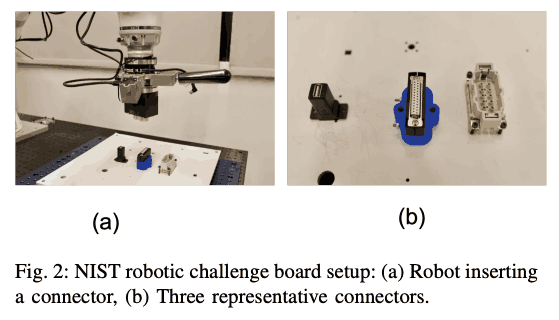## technique

The overall picture of the method is shown in the figure below. The flow is in the following order.

1. Collect Human demonstrations and store them in the replay buffer.
2. (Optional) If an image is included in the input of RL, pre-train the features of the image using DEMONSTRATION.
3. Run the current policy in your environment and collect data
4. If necessary, the human performs on-policy correction to correct the robot's behavioral errors.
5. (Optional) Do Curriculum Learning
6. Learning Actor and Critic asynchronously to data collection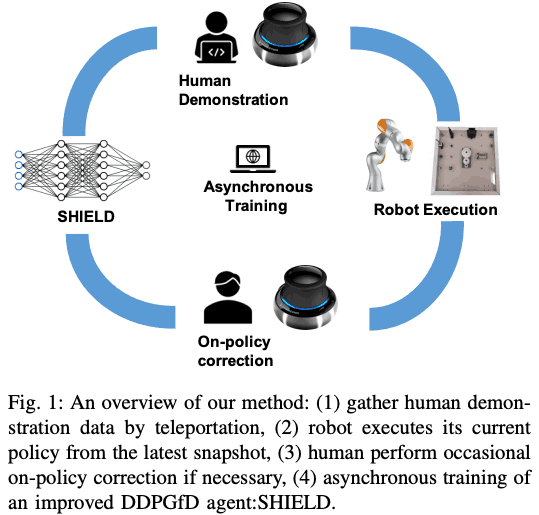### Reinforcement learning from demonstration

To train RL agents, we need to define rewards, and it is very time-consuming to set up a shaped reward function, which requires a detailed definition of the reward function. On the other hand, the sparse reward is easy to define but difficult to explore. In this paper, we propose a new method to solve this problem. In this paper, to use this demonstration, we use a method called Deterministic Policy Gradient from Demonstration (DDPGfD), mainly by modifying the red letters in the following algorithm to the original DDPGfD The following algorithms are modified from the original DDPGfD.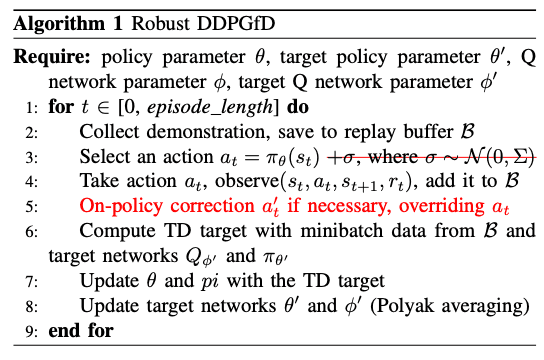The more detailed changes are as follows

1. The Gaussian noise added to the actor-network is eliminated and the deterministic policy is adopted. This is because the stochastic policy may not be able to pass efficiently through a narrow path such as an insertion task due to noise.
2. Instead of using a Prioritized replay buffer, all samples in the replay buffer have the same importance.
3. Introduce curriculum learning by gradually making task and action space more difficult.
4. When the robot is about to leave the task-solving operation, a human moves the robot with a controller to perform on-policy correction to override the action of the policy.

### Relative Coordinates and Goal Randomization

To prevent the policy from overlearning for a specific absolute coordinate, in this paper, we do not give the pose information of the robot in the base frame as the input of the policy, but the pose information relative to the pose at the time of resetting the robot as the input. In this paper, the pose information of the robot in the base frame is given as input to the policy. From the robot's point of view, this can be regarded as moving the goal of the task, i.e., the position of the socket in this paper, and has the advantage that the robot can learn for various goals without moving the goal position every time.

### Pre-train visual features

In a previous paper, we successfully solved the USB insertion task by learning a policy from images, but it took about 8 hours to achieve 80% success rate. Therefore, in this study, we train VAE using previously collected demonstrations and additionally collected data, and pre-train the image features. Then, we fix the weight of the pre-trained encoder and use it to learn the policy. In this way, we can learn the policy more efficiently.

### Multi-modal policies

Finally, let us talk about the policy input. In this paper, we tried the following four patterns
(a) Only information of robot's pose
(b) Pose information + wrist force/torque wrist force/torque
(c) pose information + wrist force/torque + wrist camera image
(d) wrist force/torque + wrist camera imge.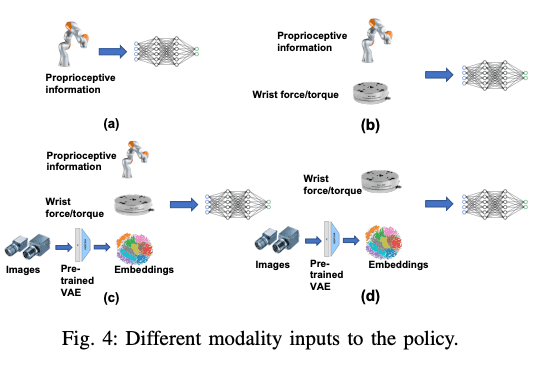## experiment

In this paper, we evaluate the method on three tasks; NIST board insertion tasks, moving target HDMI insertion, and key-lock insertion.

### NIST board insertion

The task is set up as shown in the figure below, with three different types of inputs; (1) proprioceptive information (pose information), (2) proprioceptive information + wrist force/torque, and (3) proprioceptive information + wrist force/torque + vision.We evaluated the success rate of the learned policy by adding noise to the robot's position as in training. We also evaluated the success rate when the tool center point (TCP) of the robot was 45 degrees away from the original orientation along the z-axis. In addition, to evaluate the versatility of the policy with the trained image as input, we evaluated how well the policy could be solved when the NIST board was manually moved during evaluation.

In this experiment, we used the Kuka iiwa arm, which was controlled by the end-effector Cartesian velocity using an impedance controller. If you are interested in the detailed method of the Vendor's approach, please refer to the paper.

Even this preprogrammed vendor's approach can solve the task. However, what is important here is whether the generalization performance of the vendor's approach is high, that is, whether it is robust against perturbation and so on. The following graphs show the generalization performance of the proposed method and the vendor's approach, and the generalization performance of the proposed method with RL is higher than that of the vendor's approach.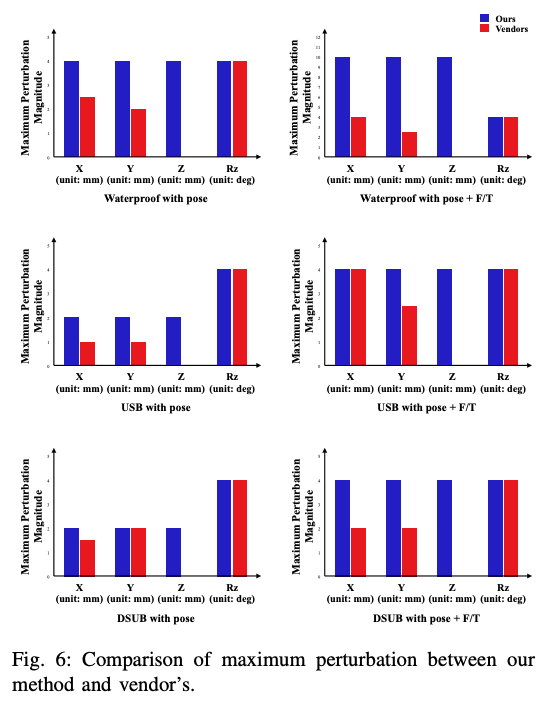The following figure shows the success rate of each modality for each connector (Waterproof, USB, DSUB), and shows that we achieved a high success rate of 13069 successes (99.8%) out of 13096 trials.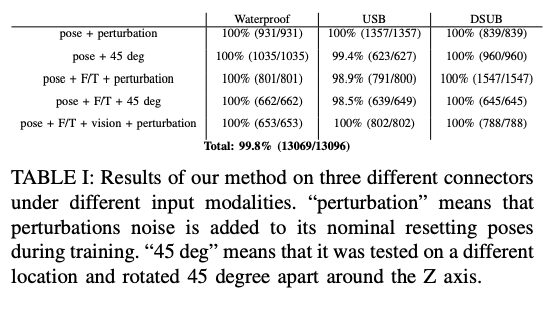### Dynamic Insertion

In this experiment, we tested whether the proposed method can quickly adapt to the motion of the NIST assembly board and solve the task when the NIST assembly board is moved by a human hand as shown in the figure below with an image as an input. As a result, we achieved a success rate of 95% out of 50 trials. The main reason for the failure was that the robot could not adapt to the movement of the NIST assembly board which was moved by hand because it was too fast.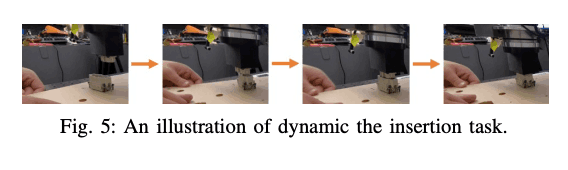### Moving HDMI Insertion

In the previous experiments, we confirmed whether the proposed method is robust or not, but the cycle time (production speed) is important in a real factory. In this experiment, we compared the cycle time between the HDMI insertion task using two robots and a human inserting the HDMI connector into the socket held by the robot (see the figure below). In this task, the IIWA robot has the HDMI connector and the Sawyer arm (left) has the HDMI socket. The Sawyer arm randomly moves in a circular motion in a plane perpendicular to the insertion direction, and the IIWA robot is tasked with inserting into it.

However, it is very difficult to learn this task from scratch, so we conducted curriculum learning using two types of curriculum: task space curriculum and action space curriculum.

In the Task space curriculum, we started by fixing the initial position and movement of Sawyer, and gradually increased the difficulty of the task by adding perturbation to the initial position and increasing the speed of the circular movement. Finally, we increased the range of the initial position of the socket to -+6 cm and the speed to 2.5 cm/s.

In the action space curriculum, if the action space of the policy is made largely from the beginning, there is a risk that the robot may collide with it and break. space exponentially. By doing so, we were able to increase the reliability of the policy.

As a result, the agent achieved a 100% success rate out of 1637 trials, and the average insertion time was 1093ms. On the other hand, the average cycle time of a human solving the task with HDMI was 1041ms, which confirms that the cycle time of the proposed method is comparable to that of a human.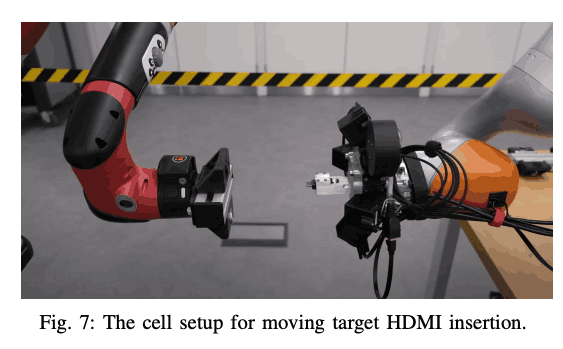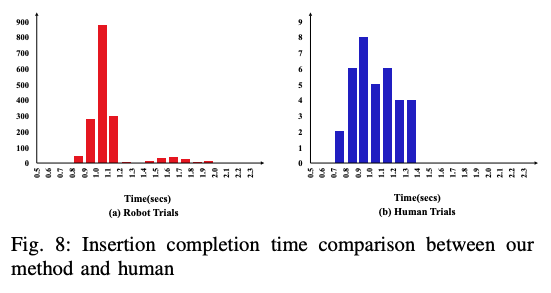### Key-lock insertion

In the previous experiments, we have used objects of a certain size and material, but in this experiment, we have used a key that is commonly used at home (see the figure below). The purpose of this experiment is to confirm whether the insertion task can be solved when the surface of the insertion part is small and the policy has to search a long distance by increasing the perturbation. In this experiment, the initial position always starts at 5cm away from the key (z-axis), and the x- and y-axis are randomly sampled and determined. In this experiment, proprioceptive information and wrist force/torque were used as input for the policy to be learned.

In this experiment, we conducted curriculum learning as in the previous experiment, and increased the difficulty of the task by starting with $b=0$ and gradually increasing it until it finally reached $b=0.5$, when we assumed that we would randomly sample between $-b$ and $b$ concerning the X/Y axis.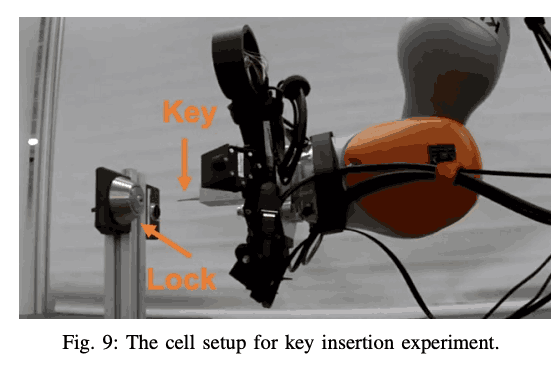The final experimental results are shown below. The results shown in the figure below show that we can achieve a relatively high success rate even for small objects such as keys.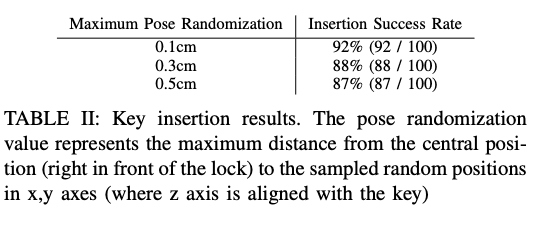## summary

In this study, we used the NIST assembly benchmark to evaluate how well the RL can solve the industrial insertion task by increasing the number of trials. However, there are various problems such as the need to fix the connector to the robot and the need to learn the policy for each connector. Therefore, we think that this is a problem that needs to be studied further in the future.